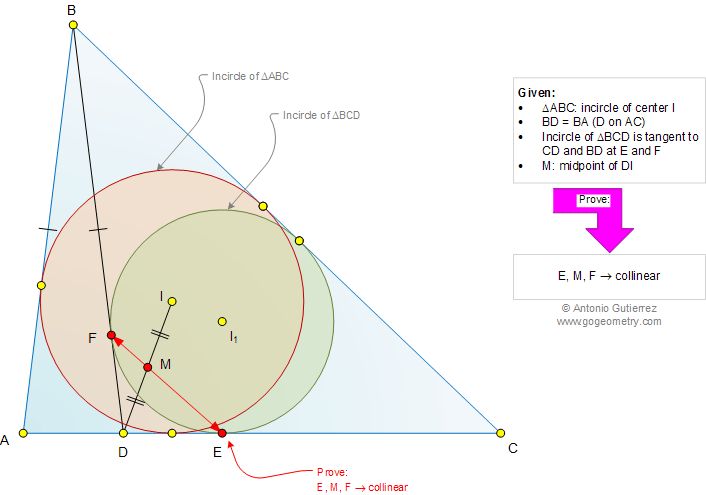# Geometry Problem 991: Triangle, Incircle, Tangency Points, Isosceles, Midpoint, Collinearity, Congruence, Circle. Level: School, College

< PREVIOUS PROBLEM  |  NEXT PROBLEM >

 The figure shows a triangle ABC of incenter I and BD = AB (D on AC). The incircle of triangle BCD is tangent to CD and BD at E and F, respectively. If M is the midpoint of DI, prove that points E, M, and F are collinear.Home | Search | Geometry | Problems | All Problems | Open Problems | Visual Index | 10 Problems | Problems Art Gallery | Art | 991-1000 | Triangles | Isosceles Triangle | Incircle | Circle | Circle Tangent Line | Midpoint | Collinear Points | Email | Solution / comment | By Antonio Gutierrez